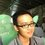# Expected chain

## Background

Audition Online is a music game where players compete to gain the most number of points in a given song. In each song, there are a certain number of bars - in each bar, a player has to execute a certain sequence of keystrokes and press spacebar on the 4th beat. A judgment is made based on how accurately the spacebar is pressed - from best to worst, "perfect", "great", "cool", "bad", and "miss". When a player does 2 or more "perfects" consecutively, he will get bonus points for doing so. Within a song, the largest number of consecutive "perfects" a player gets is called his "chain".

## Problem

A song has $n$ number of bars. Assume that a given player has a constant probability of getting a "perfect", $p$, on each bar. The chain for a particular song (largest number of consecutive "perfects") is denoted as $c$.

What is the expected value of $c$ in terms of $n$ and $p$?

Add-on: What is the probability of getting a particular $c$ in terms of $n$ and $p$? (In essence, what is the probability distribution of $c$?)

## Checks

A valid solution must minimally satisfy the following cases:

We know that given $p=1$ (i.e. a completely perfect player), $c=n$.

We also know that given $p=0$ (i.e. a completely horrible player), $c=0$.

A song with $n=1$ basically has only one bar, hence $c=p$.

A song with $n=2$ has two bars and the expected value of $c$ can be trivially calculated to be $2 p$.

## Details and assumptions

• A gameplay of P-G-P-P-G-G-G-P-G-P-P-P-P-P-G-G-P-G counts as having a chain of 5 (P is "perfect", G is "great").
• For simplicity, "greats", "cools", "bads" and "misses" are treated as the same thing. In reality, a "miss" on one bar will forfeit the next bar, hence $n$ may not be treated as a constant.Note by Louie Tan Yi Jie
7 years, 9 months ago

This discussion board is a place to discuss our Daily Challenges and the math and science related to those challenges. Explanations are more than just a solution — they should explain the steps and thinking strategies that you used to obtain the solution. Comments should further the discussion of math and science.

When posting on Brilliant:

• Use the emojis to react to an explanation, whether you're congratulating a job well done , or just really confused .
• Ask specific questions about the challenge or the steps in somebody's explanation. Well-posed questions can add a lot to the discussion, but posting "I don't understand!" doesn't help anyone.
• Try to contribute something new to the discussion, whether it is an extension, generalization or other idea related to the challenge.

MarkdownAppears as
*italics* or _italics_ italics
**bold** or __bold__ bold
- bulleted- list
• bulleted
• list
1. numbered2. list
1. numbered
2. list
Note: you must add a full line of space before and after lists for them to show up correctly
paragraph 1paragraph 2

paragraph 1

paragraph 2

[example link](https://brilliant.org)example link
> This is a quote
This is a quote
    # I indented these lines
# 4 spaces, and now they show
# up as a code block.

print "hello world"
# I indented these lines
# 4 spaces, and now they show
# up as a code block.

print "hello world"
MathAppears as
Remember to wrap math in $$ ... $$ or $ ... $ to ensure proper formatting.
2 \times 3 $2 \times 3$
2^{34} $2^{34}$
a_{i-1} $a_{i-1}$
\frac{2}{3} $\frac{2}{3}$
\sqrt{2} $\sqrt{2}$
\sum_{i=1}^3 $\sum_{i=1}^3$
\sin \theta $\sin \theta$
\boxed{123} $\boxed{123}$

Sort by:

A partial result. If the chain length, $c$, satisfies $n>c>\frac{n}{2}$, then the probability of the maximal chain being of length $c$ is

$P_n(c)=2p^c(1-p)+(n-(c+1))p^c(1-p)^2$.

Where the first term is due to the chains being right at the start or end, and the second term due to the chain being in the middle. Obviously $P_n(n)=p^n$.

For $c \le \frac{n}{2}$, it's harder as previously we didn't have to worry about what was happening in any bars other than those in chain and the one or two bars surrounding it, as the chain would always be the longest chain regardless. It's fairly easy to represent as a sum, but simplifying it would be problematic.

- 7 years, 9 months ago